# Multi Armed Bandit Problem¶

An introduction to multi-armed bandits

• toc: true
• categories: [AI]
• image: images/chart-preview.png

## Problem Description¶

Imagine you are at a casino, and you have N slot machines to play, each slot machine gives rewards according to a fixed probability distribution. What strategy should you play with to maximise your total reward ?

This problem is known as Multi Armed Bandit problem.

In :
# Importing numpy for math, and matplotlib for plots
import matplotlib.pyplot as plt
import numpy as np
%matplotlib inline


## Arms¶

An arm when pulled, gives a random number from a normal distribution with fixed mean(mu) and deviation(sigma). When pulled many times the frequency of the rewards look like this:X axis is the magnitude of reward
Y axis is it's frequency.

The Arm class provides an arm with these properties.

In :
class Arm:

def __init__(self, mu=None, sigma=None):
if mu is None:
self.mu = np.absolute(np.random.uniform())
else:
self.mu = mu

if sigma is None:
self.sigma=np.absolute(np.random.uniform())
else:
self.sigma = sigma

def pull(self):
reward = np.random.normal(self.mu, self.sigma, 1)
return reward

def get_arms(k):
# returns a list of arms
arms = []
for i in range(k):
arms.append(Arm())
return arms


## Agents¶

An agent here is a player who pulls arms to play. It has a policy, which is a list of probabilities associated with each arm.

The agent class makes designing agents fast. The object is initialised with arms and whether it should play all arms once as part of the initialisation.

Features provided by this class:
Attributes:

• expectations[i]: gives the expected reward on playing arm[i]
• times_played[i]: gives the number of times the agent has played arm[i]
• N = Total number of times agent has played
• reward_history : list of rewards earned by the agent
• choice_history : list of choices made by the agent

Methods:

• gamble(i): Plays for i iterations while updating it's policy.
• play(i): Pulls arm[i] and updates reward_history, N , times_played
• select_arm(): returns index of an arm by sampling probability distribution given by the policy
In :
class agent:
def __init__(self, arms, play_once=1):
self.expectations = np.zeros(len(arms))
self.times_played = np.zeros(len(arms))
self.arms = arms

self.number_of_arms = len(arms)
self.N = 0

self.reward_history = []
self.choice_history = []

if play_once == 1:
for i in range(self.number_of_arms):
self.expectations[i] = self.play(i)

def play(self, index):
reward = self.arms[index].pull()

self.times_played[index] += 1
self.N += 1

self.choice_history.append(index)
self.reward_history.append(reward)

return reward

def policy(self):
pass

def update_expectations(self, reward, index):
self.expectations[index] += (reward - self.expectations[index])/self.N

def select_arm(self):
options = range(self.number_of_arms)
i = np.random.choice(options, p=self.policy(), replace=False)
return i

def gamble(self, iterations):
for i in range(iterations):
index = self.select_arm()
reward = self.play(index)
self.update_expectations(reward, index)


### Example agents¶

To make a new agent we inherit the agent class.

Time to make some agents!

### First up: epsilon-greedy¶

This agent plays the arm with the highest expected reward with 1 - epsilon probability, and plays a random arm with epsilon probability

So
epsilon = 1 => random choices
epsilon = 0 => greedy choices

In :
class epsilon_greedy(agent):

def __init__(self, arms, play_once=1, epsilon=0.1):
super().__init__(arms, play_once)
self.epsilon = epsilon

def __str__(self):
return "Epsilon-Greedy Agent, epsilon= "+str(self.epsilon)

def policy(self):
temp = np.zeros_like(self.expectations)
temp[np.argmax(self.expectations)] = 1-self.epsilon
ans = temp + self.epsilon/self.number_of_arms
return ans


### Beta-Softmax¶

This agent plays an arm[i] with probability proportional to: e^(expected_reward(arm[i])/beta)
We normalise the whole thing by the sum over all the arms.

In :
class softmax(agent):

def __init__(self, arms, play_once=1, beta=1):
super().__init__(arms, play_once)
self.beta = beta

def __str__(self):
return "Softmax agent, beta= "+ str(self.beta)

def policy(self):
temp = np.exp(self.expectations/self.beta)
ans = temp / np.sum(temp, axis=0)
return ans


### Upper Confidence Bound (UCB1)¶

UCB1 agent plays the arm with the highest metric, where metric of arm i is : metric[i] = expected_reward[i] + sqrt(2*log(N)/times_played[i])

Note Best peformance when rewards are between 0 and 1

In :
class ucb(agent):

def __init__(self, arms, play_once=1):
super().__init__(arms, play_once)

def __str__(self):
return "UCB1 agent"

def policy(self):
temp = self.expectations + np.sqrt(2*np.log(self.N)/self.times_played)
ans = np.zeros_like(temp)
ans[np.argmax(temp)] = 1
return ans


## Metrics¶

Metric : A scalar number, makes comparison easier.
To compare the performance of our agents we can use these metrics

• avg_reward[i] : this gives the average reward till i+1 iteration.
• max_reward : this tells us the maximum expected reward

• euclid_distance : we can think of as learnt policy and optimal policy as vectors and compute the distance between them , smaller is better

• cosine_simmilarity : compute the cos(q) between the policies. larger is better
In :
def maxreward(arms):
#Max rewards
a= [arm.mu for arm in arms]
return max(a)

def avg_reward(rewards):
ans = []
ans.append(rewards)
for i in range(1,len(rewards)):
ans.append(ans[i-1]+rewards[i])
for i in range(len(ans)):
ans[i]/=i+1
return ans

def cosine_similarity(a,b):
temp = a*b
temp/=(euclid_distance(a)* euclid_distance(b))
return np.sum(temp, axis=0)

def euclid_distance(a):
return np.sqrt(np.sum(a*a, axis=0))


### Test¶

This function takes a list of agents and the number of iterations. Makes each agent play, and prints its metrics.

In :
def test(agents, iterations):
for agent in agents:

agent.gamble(iterations)

temp = [ arm.mu for arm in levers]
optimal_policy = np.zeros_like(agent.expectations)
optimal_policy[temp.index(max(temp))] = 1

avg_rewards_earned = avg_reward(agent.reward_history)

print(agent)
print("maximum possible reward:", maxreward(levers))
print("average reward:", avg_rewards_earned[-1])
print("cosine similarity" ,cosine_similarity(agent.policy(), optimal_policy))
euclid_norm = euclid_distance(agent.policy()-optimal_policy)/len(optimal_policy)
print("euclidian norm ",euclid_norm)

plt.plot(avg_rewards_earned)
plt.ylabel('Average Reward')
plt.xlabel('Iteration')
plt.show()
print("\n")

# print("optimal policy:" , optimal)
# print("learnt policy:" ,agent.policy())

# plt.scatter(range(len(agent.choice_history)),y=agent.choice_history)
# plt.title("Choices")
# plt.xlabel("time")
# plt.ylabel("arm")
# plt.show()
# print("\n")


In :
levers = get_arms(10)

agents = [
epsilon_greedy(levers, epsilon=1),
epsilon_greedy(levers, epsilon=0),
softmax(levers, beta=0.1),
ucb(levers)

]

In :
plt.plot([ arm.mu for arm in levers] )
plt.title("distribution of expected value of arms")

Out:
Text(0.5, 1.0, 'distribution of expected value of arms')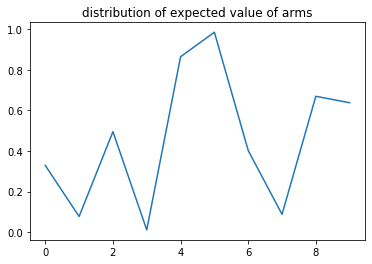In :
test(agents, 5000)

Epsilon-Greedy Agent, epsilon= 1
maximum possible reward: 0.9851042878107023
average reward: [0.47962497]
cosine similarity 0.3162277660168379
euclidian norm  0.09486832980505139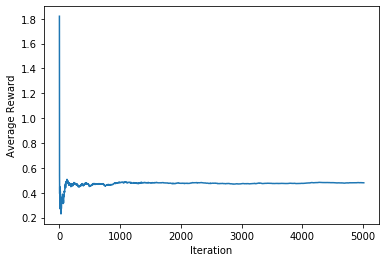Epsilon-Greedy Agent, epsilon= 0
maximum possible reward: 0.9851042878107023
average reward: [0.98686237]
cosine similarity 1.0
euclidian norm  0.0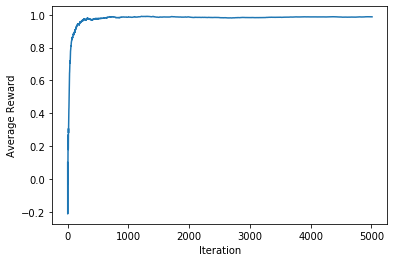Softmax agent, beta= 0.1
maximum possible reward: 0.9851042878107023
average reward: [0.91348264]
cosine similarity 0.9992727823574249
euclidian norm  0.008915931500017809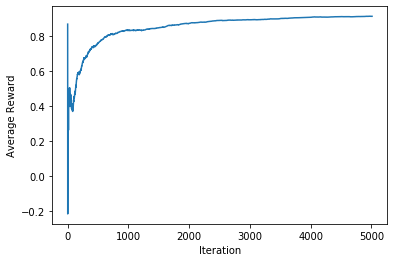UCB1 agent
maximum possible reward: 0.9851042878107023
average reward: [0.89258379]
cosine similarity 0.0
euclidian norm  0.1414213562373095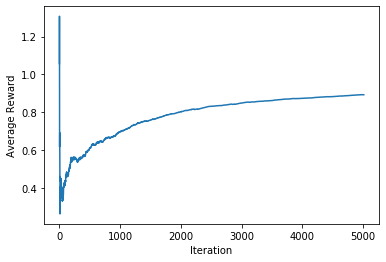### Experimental stuff:¶

Below are a few agents I wrote for fun.

In :
class softmax_with_exponentiation(agent):

def __init__(self, arms, play_once=1, beta=1, exp=1):
super().__init__(arms, play_once)
self.beta = beta
self.exp = exp

def policy(self):
temp = np.exp(self.expectations/self.beta)
ans = temp / np.sum(temp, axis=0)
ans = ans**self.exp
ans /= np.sum(ans, axis=0)
return ans

class softmax_with_reccurence(agent):

def __init__(self, arms, play_once=1, beta=1):
super().__init__(arms, play_once)
self.old_policy = np.ones_like(self.expectations)/self.l
self.beta = beta

def policy(self):
temp = np.exp(self.expectations/self.beta)
new_policy = temp / np.sum(temp, axis=0)

result = np.multiply(new_policy, self.old_policy)
result /= np.sum(result, axis=0)
self.old_policy = result

return result

class greedy_with_reccurence(agent):
# alpha = number < 1; will sum over a number of observations and will keep
# osiclating.
# alpha = N will allow the algo to converge to an arm, greedy doesn't
# really need this, kind of always give one answer.

def __init__(self, arms, play_once=1, alpha=1):
super().__init__(arms, play_once)
self.old_policy = np.ones_like(self.expectations)
self.alpha = alpha

def policy(self):
new_policy = np.zeros_like(self.expectations)
new_policy[np.argmax(self.expectations)] = 1

new_policy = (1-self.alpha)*new_policy + self.alpha*self.old_policy

new_policy /= np.sum(new_policy, axis=0)
self.old_policy = new_policy

return new_policy

# class magic(agent):
#    def __init__(self, arms, play_once=1, exp=1):
#        super().__init__(arms, play_once)
#        self.old_policy = np.ones_like(self.expectations)/self.l
#        self.exp = exp
#
#    def policy(self):
#        new_policy = f(old_policy, g(expectations))